# Integers + multiplication - math problems

#### Number of problems found: 31

• Paulownia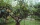Seed germination is 80%. Of the sprouted plants, 8% subsequently die. How many plants does a gardener grow from 1,500 seeds?
• Francesca 2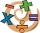Francesca applied the steps below to find the product of (-1.2)(-9.4). Step 1: (-1.2)(-9.4) = (-9.4)(-1.2) Step 2:                   = (-9.4)(-1) + (-9.4)(-0.2) Step 3:                   = (9.4) + (1.88) Step 4:                   = 11.28 Which step showsAdd six to twelve, then divide the sum by four. Divide the quotient by negative nine and then multiply by twenty-four. What is the result?
• Multiplication negativesJosh says that (4)(-7)(-1) is equal to (4)(7). Evangelina says (4)(-7)(-1) is equal to (-28)(-1) . Who is correct? Explain your reasoning.
• A bottle 2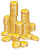A bottle contain 255 coins 1/3 of the coins are £1 cons 110 of the coins are 50p coins the rest of the coins are 20p coins. What is the total value of the coins contained in the bottle.
• Chocolate chipOut of 28 cookies, 3/4 are chocolate chip. How many cookies are chocolate chip?
• The number 4Two-thirds of a number is negative six. Find the number.
• The elevation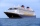The elevation of a sunken ship is -120 feet. Your elevation is 5/8 of the ship’s elevation. What is your elevation?
• Evaluate expressionIf x=2, y=-5 and z=3 what is the value of x-2y
• Evaluate - order of opsEvaluate the expression: 32+2[5×(24-6)]-48÷24 Pay attention to the order of operation including integers
• Three-digit integersHow many three-digit natural numbers exist that do not contain zero and are divisible by five?
• Expression plus minusEvaluate expression: (-1)2 . 12 – 6 : 3 + (-3) . (-2) + 22 – (-3) . 2
• DozenWhat is the product of 26 and 5? Write the answer in an Arabic numeral. Add up the digits. How many of this is in a dozen? Divide #114 by this
• SimplifySimplify expression - which expression is equivalent to: 3(m + 2) − 4(2m − 9)
• Progression-12, 60, -300,1500 need next 2 numbers of pattern
• Expression 6Evaluate expression: -6-2(4-8)-9
• Brackets 2Add parenthesis to make true: 5-2×6-4+2=5
• Product of the sum and differenceCalculate the product of the sum and difference of numbers -7 and -2.
• MO Z6-6-1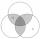Write integers greater than 1 to the blanks in the following figure so that each darker box was a product of the numbers in the neighboring lighter boxes. What number is in the middlebox?
• Value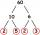Determine the value of the following exspressions: a) (23-25)·(4-5) b) (97-123):(18+8)

Do you have an exciting math question or word problem that you can't solve? Ask a question or post a math problem, and we can try to solve it.

We will send a solution to your e-mail address. Solved examples are also published here. Please enter the e-mail correctly and check whether you don't have a full mailbox.

Integers - math problems. Multiplication Problems.